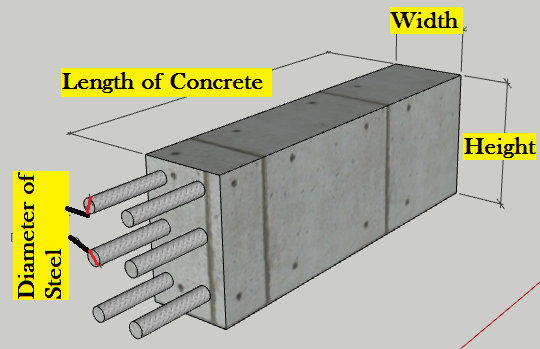# Concrete Weight Calculator

## Concrete Weight Calculator## How to use Concrete Weight Calculator?

• Choose the types of concrete and input the dimension of concrete from the input section of the calculator.
• Click on calculate button to get the total weight of concrete.
• Enjoy the Calculator.

## How to calculate Concrete weight?

Steps:-

First calculate the volume of concrete with the help of the length, width and height of the structure.

Then multiply the volume by their density to get the total weight of concrete.

Let, Total volume of concrete be 5 m3. Calculate the weight of concrete.

We have, density of Plain Cement Concrete = 2400 Kg/m3

The, Weight of concrete = Volume x Density = 5 x 2400 = 12000 Kg.

Hence, in this way we can calculate the weight of concrete.

Note* :-

Density of Reinforced cement Concrete = 2500 Kg/m3

Density of Mortar = 2162 Kg/m3

## How to calculate unit weight of concrete?

Unit weight of concrete is also called density of concrete. The unit weight of concrete can be calculated by dividing Mass of concrete by Its volume.

i.e Unit weight of Concrete = Mass/Volume

## How do you calculate the weight of a concrete slab?

To calculate the weight of a concrete slab is very easy procedure.

Calculate the volume of Slab by multiplying the length, width and height of slab.

Now, density of plain cement concrete and Reinforced cement concrete is given as 2400 Kg/m3 and 2500 Kg/m3 respectively.

If you have PCC i.e plain cement concrete then,

Weight of Slab = Volume x 2400 Kg.

If you have RCC i.e Reinforced cement concrete then,

Weight of Slab = Volume x 2500 Kg.

Sharing Is Caring: